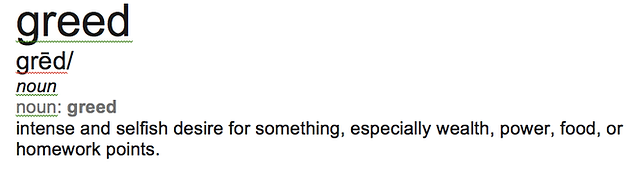## Day 61 - Lesson 6.3

##### Learning Targets
• Find probabilities involving geometric random variables.

##### Activity:This activity is based loosely on an old dice game called “GREED” — the hottest game on Dice. For the details on all the real rules, go here.

We told students that every point they earn will be added to their homework score for the chapter, so this was high stakes. All students start by standing for the first round. In each round, the teacher rolls a die. If a 1-5 shows up, all standing students earn a point. If a 6 shows up, all standing students go to 0 points and are out of the game. Before the start of each round, students have the option to quit with the number of points they have accumulated up to that round.

##### Is this a binomial distribution?

Students started by thinking that they could use their knowledge about binomial distribution to work through the activity. They realize quickly that this won’t work, because there is not a fixed number of trials. As Dan Meyer would say, we broke their tool (thus requiring learning about a new tool.) They can use their previous knowledge about probability of independent events to answer all the questions in the activity. In the debrief, we formalized this work by turning it into a formula.

##### Should I teach geometric distribution?

We have talked to many AP Statistics teacher who skip this lesson to save time. Some even claim that it is not part of the AP Exam. It is definitely included in the Content Specifications for the AP Exam. More important, we think this lesson gives students more practice with probability thinking and reasoning, which we think is worth the time.

##### An opportunity for thinking about a significance test!

In 2nd hour today, it took 21 rolls of the die until we had a 6 show up. One of the students stayed greedy until 17, earning him 17 extra credit homework points. We were so surprised by this result that we calculated the probability that a fair die would take 21 or more rolls to get a 6. The probability is 0.026. Does this provide convincing evidence that this die was unfair?